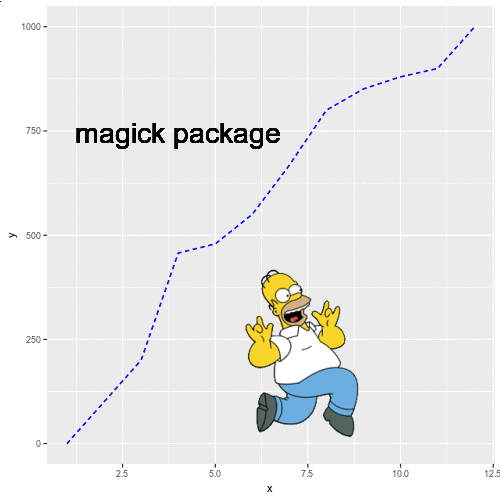# Image processing in R with magick

#### Author

Jeroen Ooms

You can import an image (PNG or JPEG) to R with the `image_read` function from the `magick` package. If you assign the result to an object then you can print it with the `print` function. Note that you can set `info = FALSE` to avoid printing the information about the image.

``````# install.packages("magick")
library(magick)

# Printing the image
print(image, info = FALSE)``````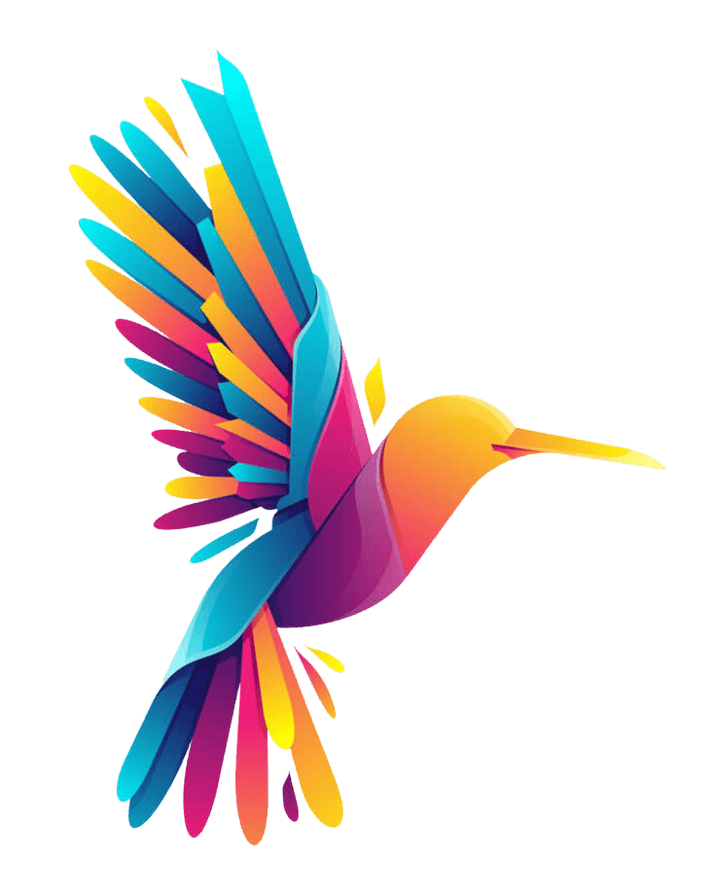Get the image details

If you want to obtain the width, height and other details about the image you can use the `image_info` function and assing the result to a variable. Then, you will be able to access each of the elements, such as the width and height of the imported image.

``````# install.packages("magick")
library(magick)

# Image details
info <- image_info(image)

# See the details
print(info)

info\$width # 250px
info\$height # 250px``````
``````  format width height colorspace matte filesize density
1    PNG   720    893       sRGB  TRUE    68794   72x72``````

With the `image_read_svg` function you will be able to import a SVG file. In this scenario, you will need to set the width and height with the corresponding arguments.

``````# install.packages("magick")
library(magick)

width = 500, height = 500)

print(image, info = FALSE)``````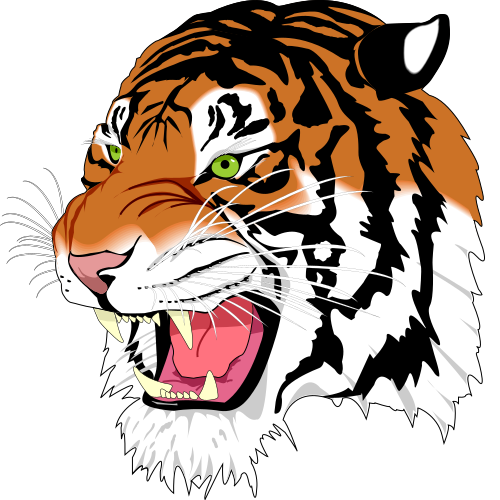You can convert an image to other formats with the `image_convert` function, specifying the desired output format in the `format` argument of the function.

Read a PDF document as image

The `image_read_pdf` function allows reading PDF documents and transforming each page into a PNG. You can choose any page or pages with the `pages` argument, but if not specified, all pages will be converted and then you will be able to see all.

``````# install.packages("magick")
# install.packages("pdftools")
library(magick)

# All pages
pdf_doc

# Page 1
pdf_doc[]

pdf_page <- image_read_pdf('https://cran.r-project.org/web/packages/calendR/calendR.pdf', pages = 1)
pdf_page``````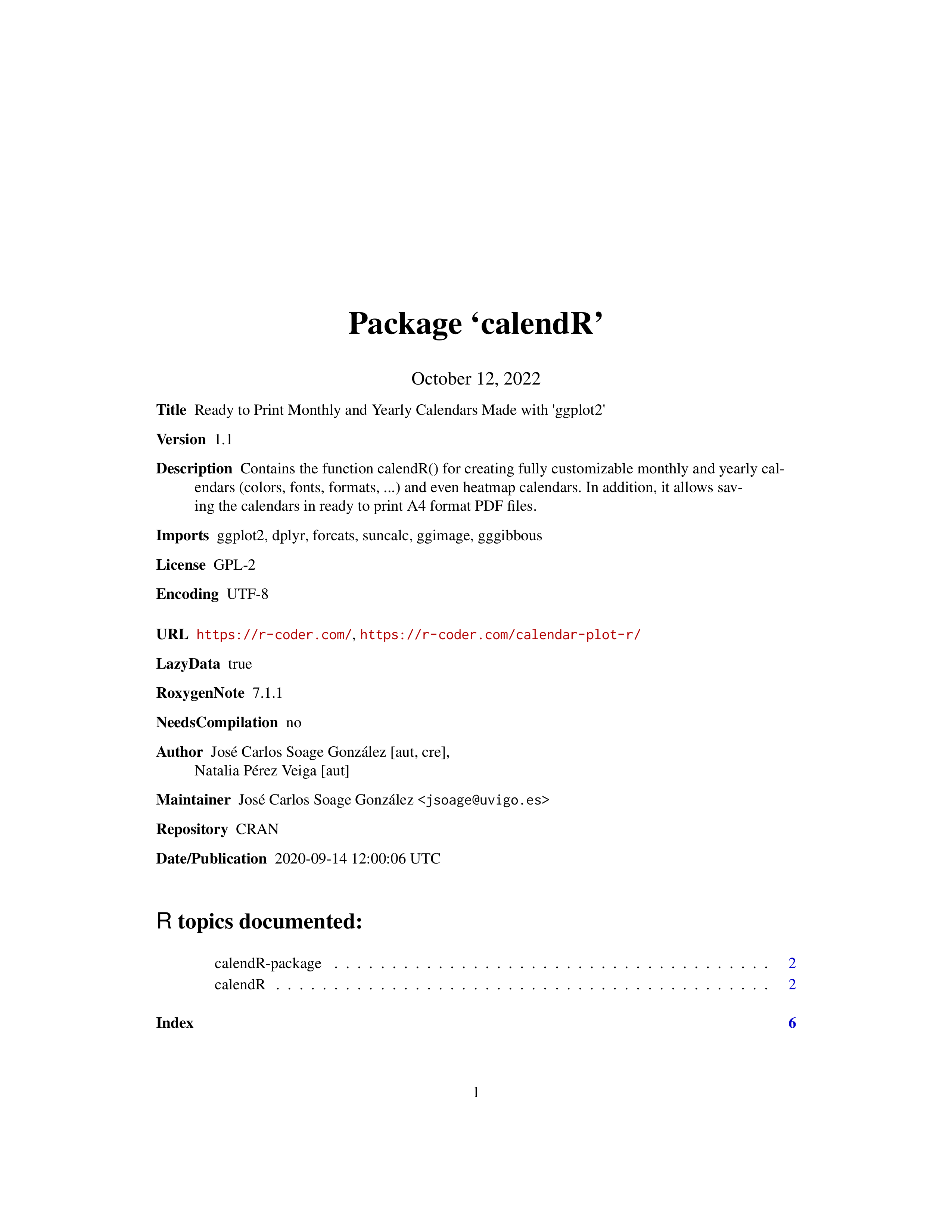It is possible to extract the text from an image with the `image_ocr` function. For instance, in the previous example you could write `cat(image_ocr(pdf_doc))` to extract the text. Note that the `tesseract` package must be installed in order to work.

There are several functions to export the images, gifs and videos created with `magick` package. These functions are: `image_write`, `image_write_gif` and `image_write_video`.

## Image editing

The magick package provides several functions to scale, crop, trim, rotate and paint images. These functions will be highlighted in the following blocks of code.

### Resize and crop

Scale the image to a specific width

If your image is too big or too small you can make use of the `image_scale` function to scale it to the desired size. By default, you can input an integer as character to scale the width of the image to that size in pixels.

``````# install.packages("magick")
library(magick)

image_scale(r, "200")``````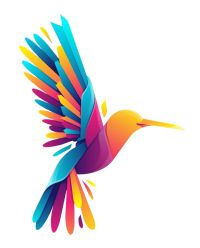Scale the image to a specific height

If you add an `x` before the number instead of width the number will be interpreted as the desired height of the scaled image.

``````# install.packages("magick")
library(magick)

image_scale(r, "x400")``````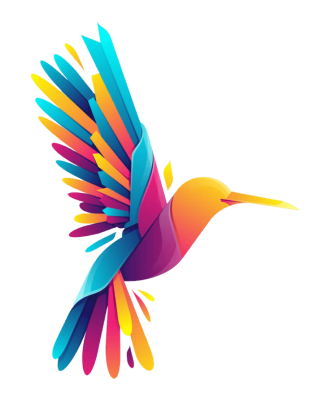Trim the margins of the image

If your image is a PNG and the transparent area is big you can use the `image_trim` function to trim the margins of the image.

``````# install.packages("magick")
library(magick)

image_trim(r)``````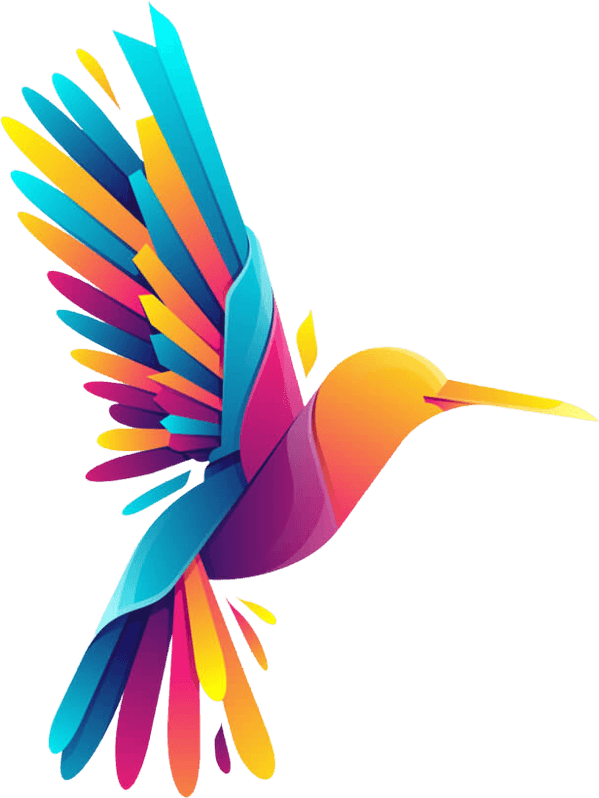Crop the image

Making use of the `image_crop` function you can crop the image. In order to crop an image you will need to specify a geometry indicating the width and height for the resulting image and where to make the crop.

``````# install.packages("magick")
library(magick)

# Width: 200px, height: 300px,
# starting at +200px from the left and 300 from the top
image_crop(r, geometry = "200x300+200+300")``````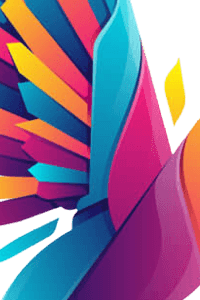### Rotate

Rotating the image

With `image_rotate` you can rotate the image the angle specified in `degrees`.

``````# install.packages("magick")
library(magick)

image_rotate(r, degrees = 45)``````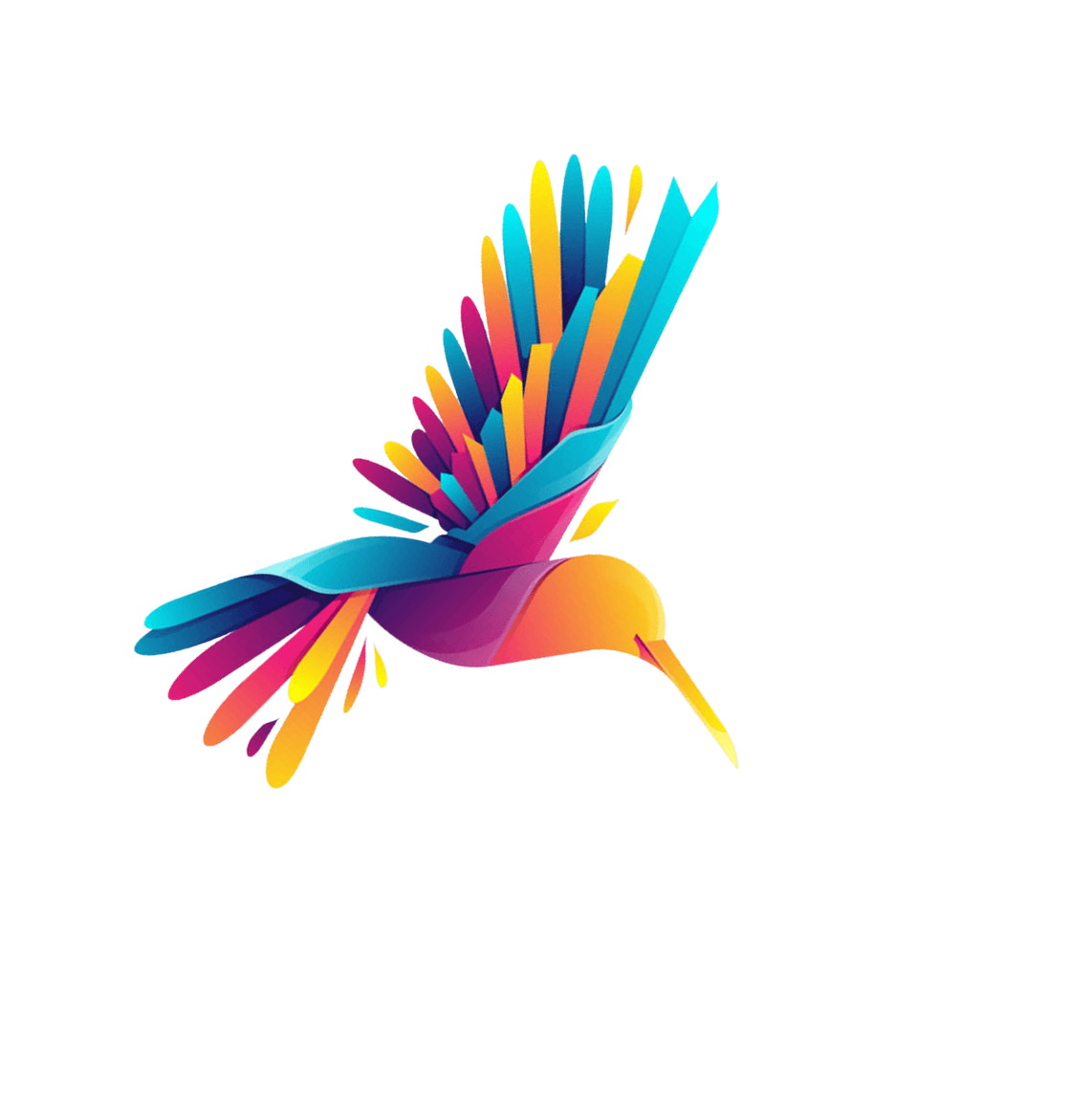Vertical flip

The `image_flip` function is a shortcut if you want to flip the image vertically instead of specifying the degrees.

``````# install.packages("magick")
library(magick)

image_flip(r)``````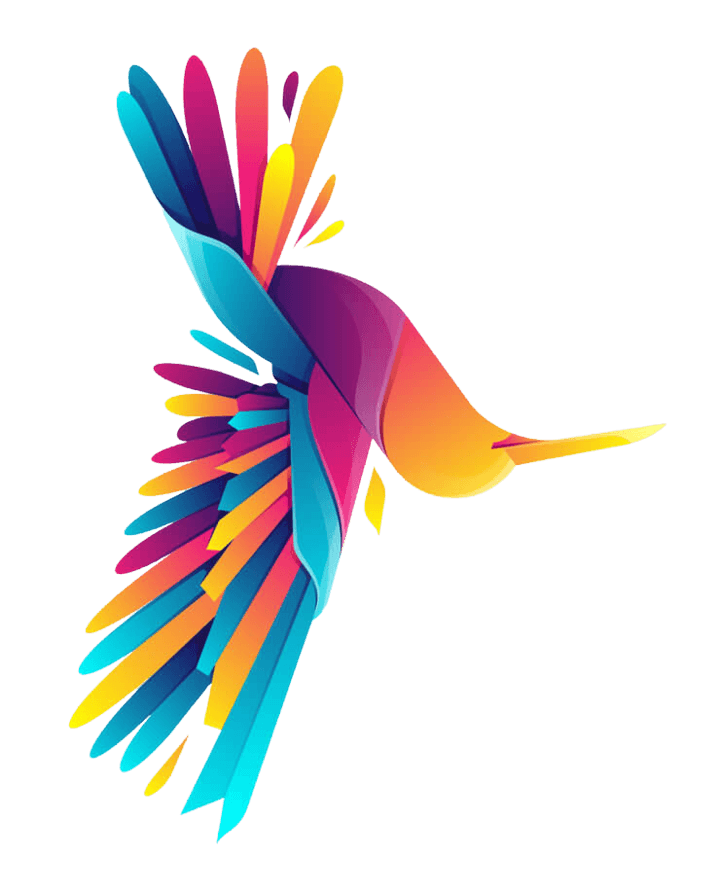Horizontal flip (mirror)

You can mirror the image (horizontal flip) with the `image_flop` function, as shown in the example below.

``````# install.packages("magick")
library(magick)

image_flop(r)``````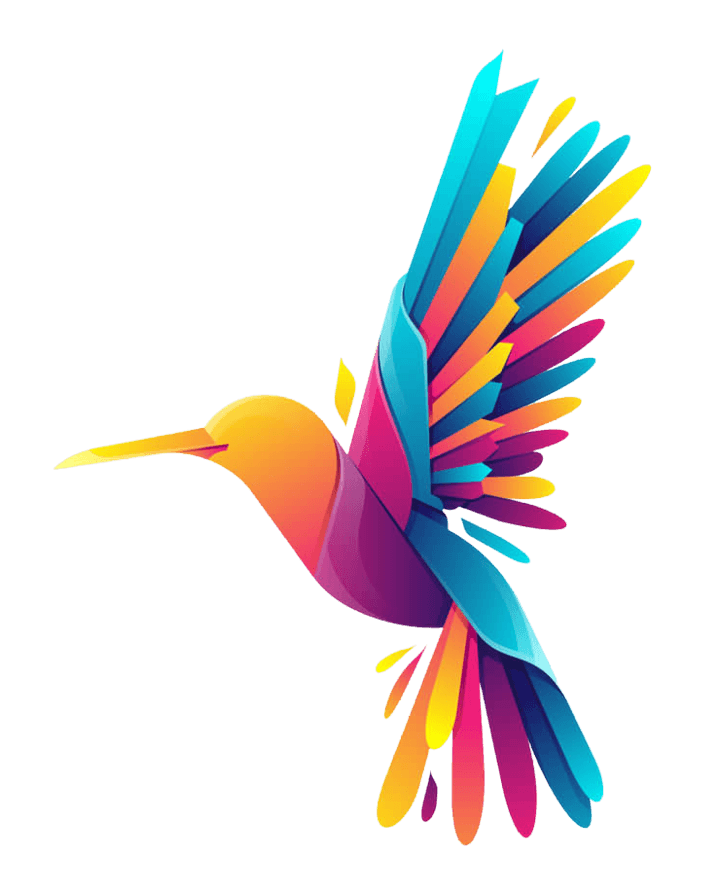### Paint

Background color

If your image is a PNG with transparent background you can set a background color with the `image_background` function. The color can be specified using the `color` argument.

``````# install.packages("magick")
library(magick)

image_background(r, color = "#cbe7ff")``````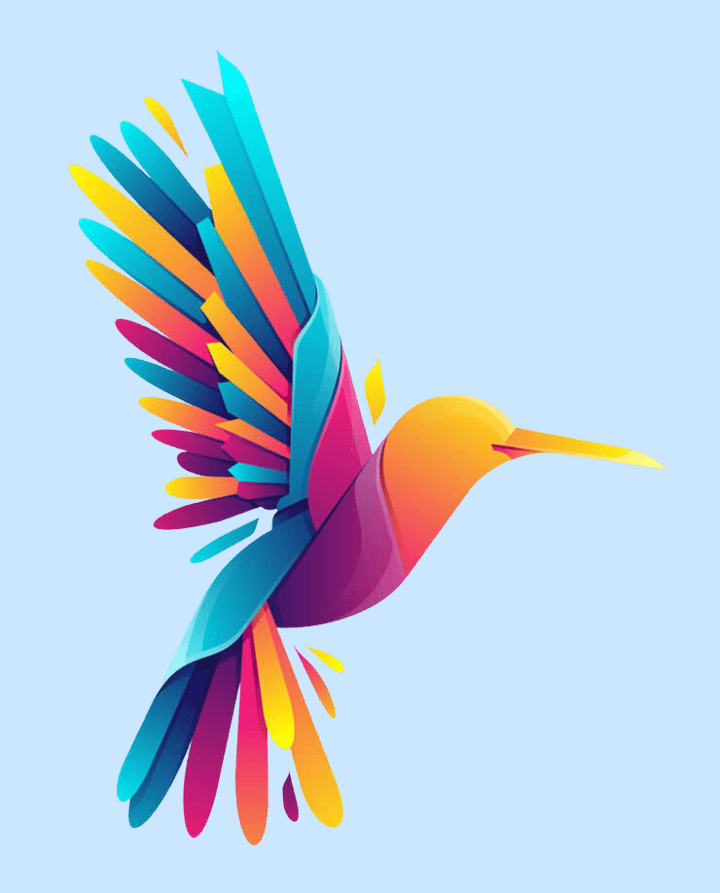You can add a border to your image with `image_border`. For that purpose, you will need to specify a color and a geometry. In the following example we are creating a border with a height of 8 pixels and width of 10 pixels. An alternative function to add a border is `image_frame`.

``````# install.packages("magick")
library(magick)

image_border(r, color = "#cbe7ff", geometry = "8x10")``````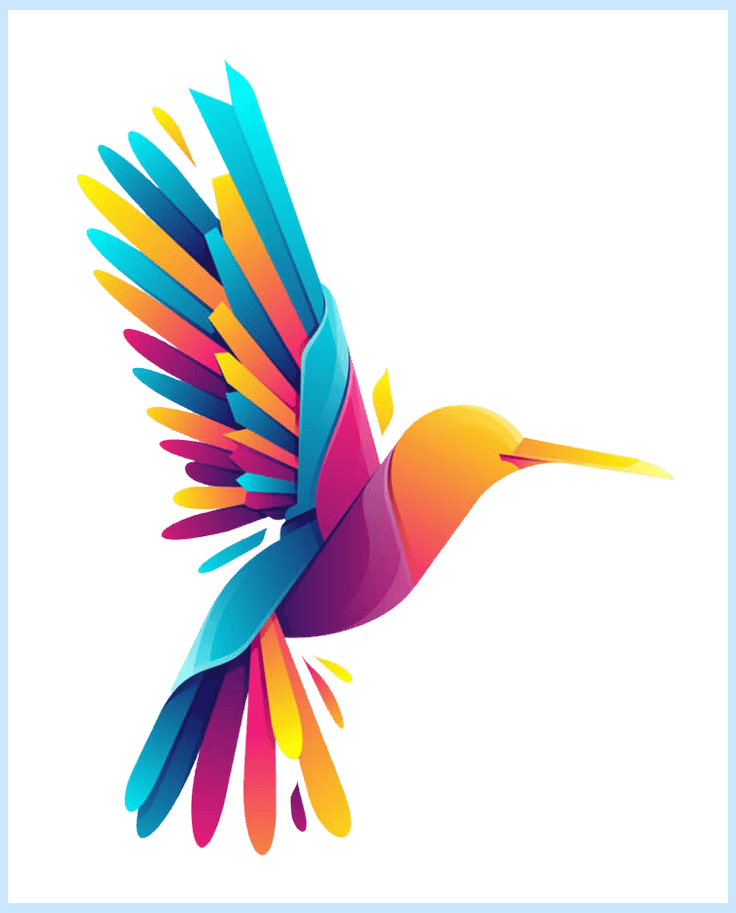Fill with color at a specific point

The `image_fill` function allows changing a specific color that exists on a point. In the following example we are changing the color to blue at `"+420+520"`, which means that the point is placed at `x = 420` and `y = 520` coordinates, but note that the image of the sample has gradient colors, so the result is not good. This function will be very useful, for instance, to change the background color of a PNG file.

``````# install.packages("magick")
library(magick)

image_fill(r, "blue", "+420+540")``````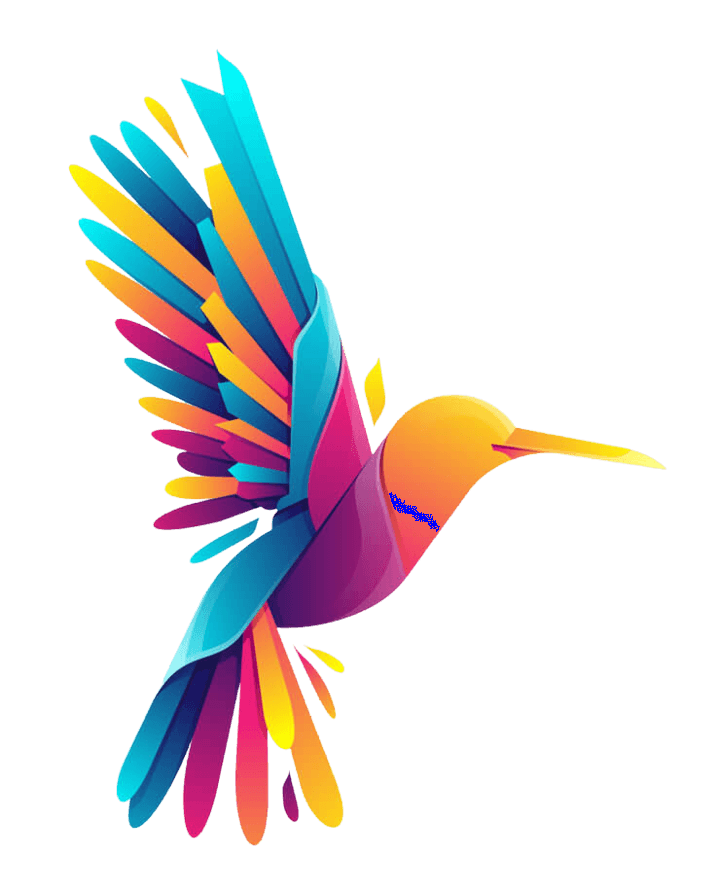With `image_annotate` it is possible to add texts over images. You just need to pass the desired image and the text and then set the size, the angle, the gravity (type `gravity_types()` for the list of available options) or locations and other styling, such as the color, stroke, weight or font family with the arguments provided by the function.

``````# install.packages("magick")
library(magick)

image_annotate(r, "Text over an image", size = 75, gravity = "center", color = "black")``````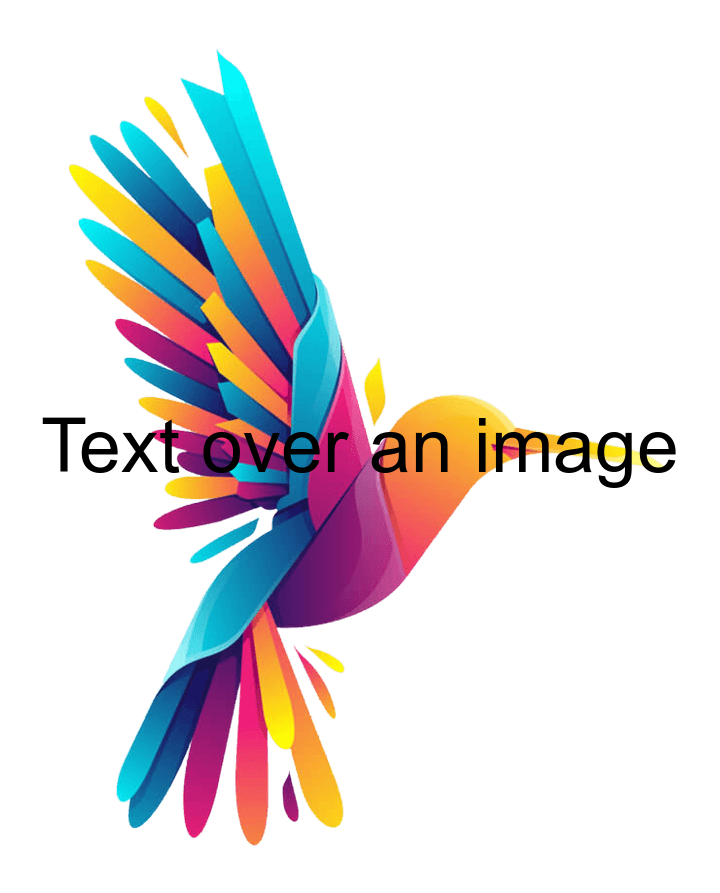Text inside a box

Note that the function also provides an argument named `boxcolor` that can be set to any color to create a box around the text.

``````# install.packages("magick")
library(magick)

image_annotate(r, "Text over an image", size = 75, gravity = "center", color = "black",
degrees = 45, boxcolor = "white")``````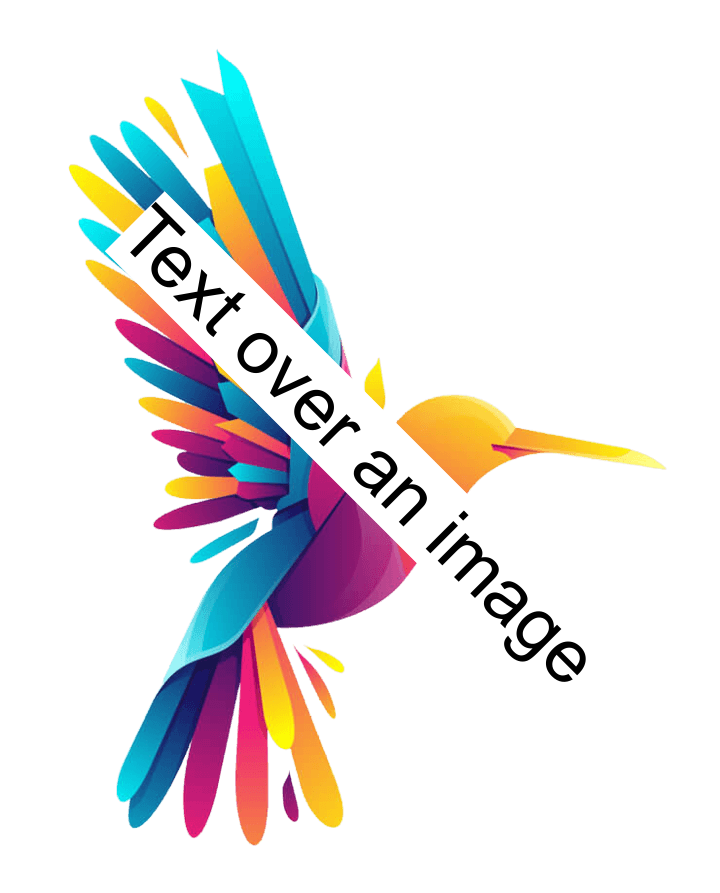## Image effects and filters

You can distort an image by adding blur to it with the `image_blur` function. This function provides two arguments named `radius` (defaults to 1) and `sigma` (defaults to 0.5) to customize the level of blur.

``````# install.packages("magick")
library(magick)

image_blur(r, radius = 10, sigma = 5)``````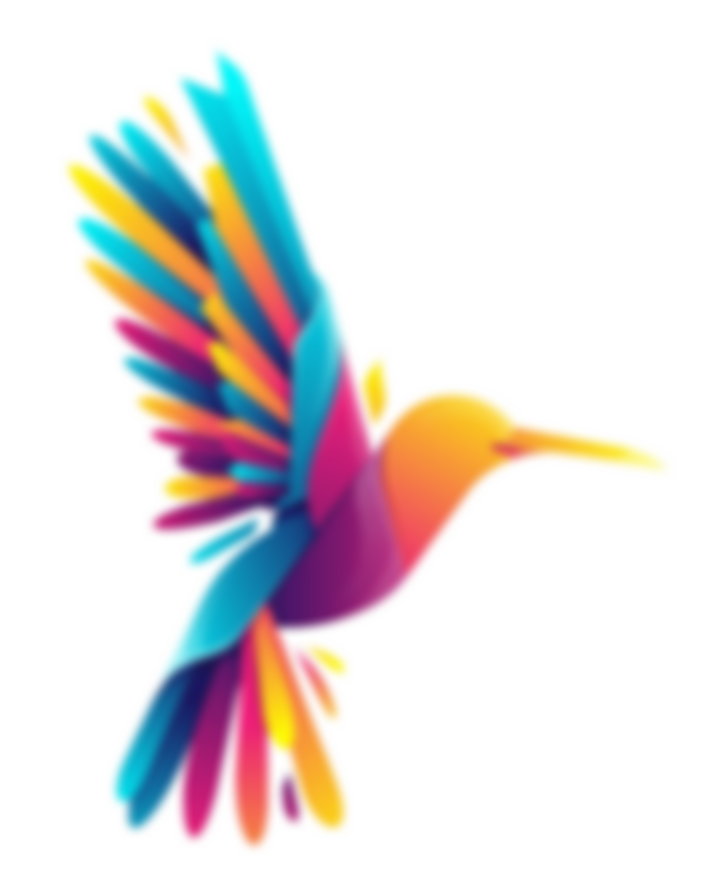The `image_noise` function allows adding noise to the image. By default, the noise is gaussian, but you can check the available noise types with `noise_types()`.

``````# install.packages("magick")
library(magick)

image_noise(r)``````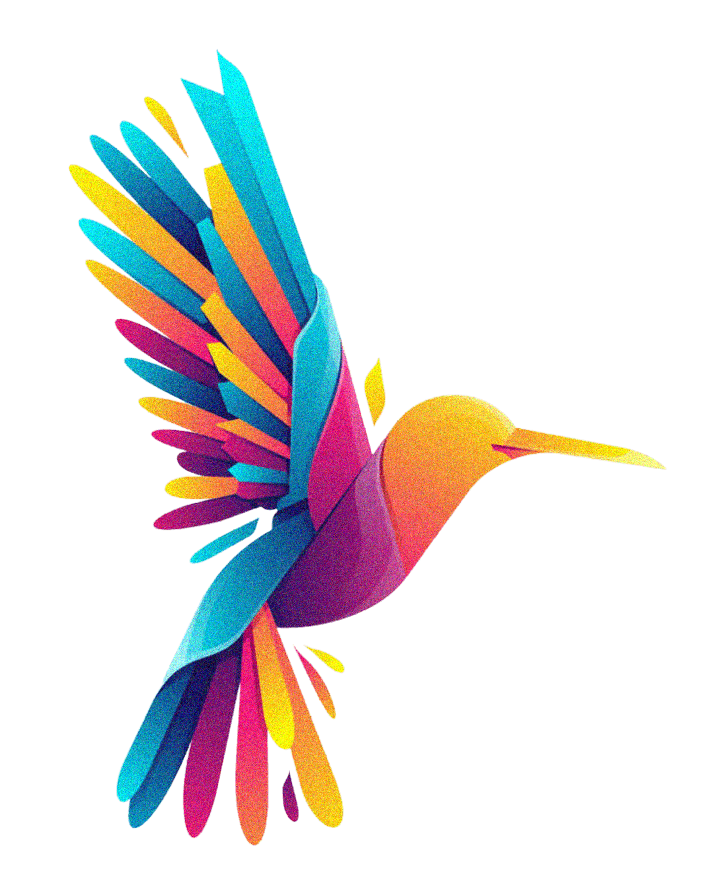Invert the color

With `image_negate` you will be able to invert the colors of the image.

``````# install.packages("magick")
library(magick)

image_negate(r)``````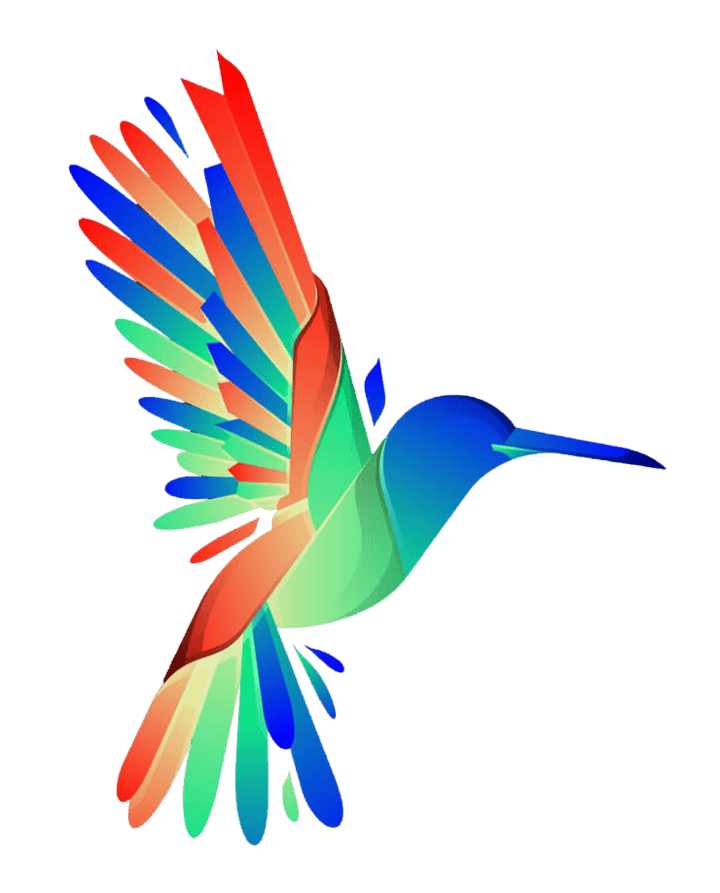Charcoal effect

The magick package also provides some effects, such as the charcoal effect with `image_charcoal`.

``````# install.packages("magick")
library(magick)

image_charcoal(r)``````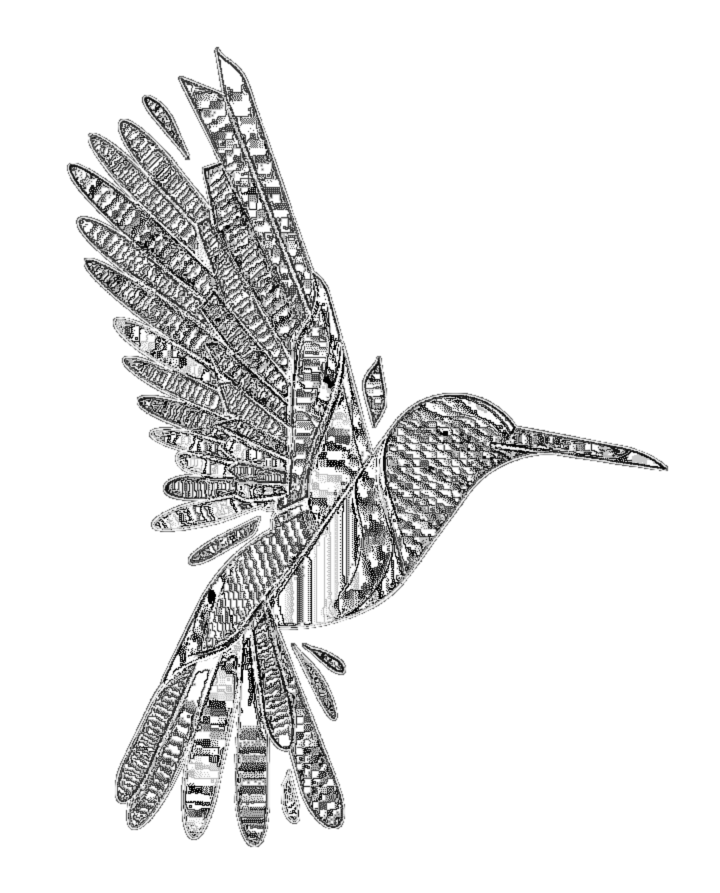Implode effect

Other effect is the implode effect, which will implode the image based on a implode factor.

``````# install.packages("magick")
library(magick)

image_implode(r, factor = 0.75)``````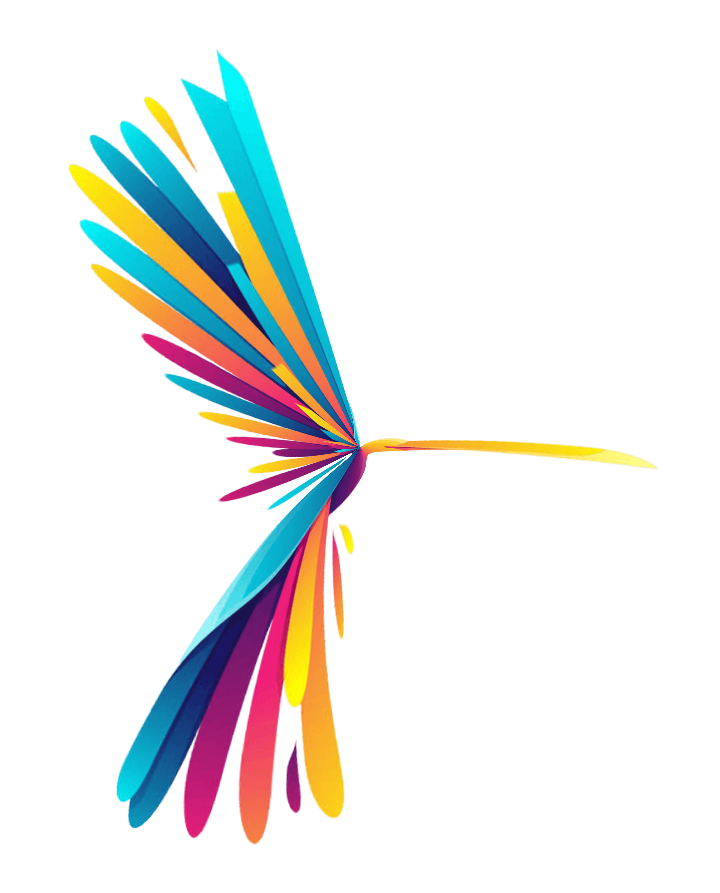Oil paint effect

The last effect we are going to highlight is the oil paint effect that can be created with the `image_oilpaint` function.

``````# install.packages("magick")
library(magick)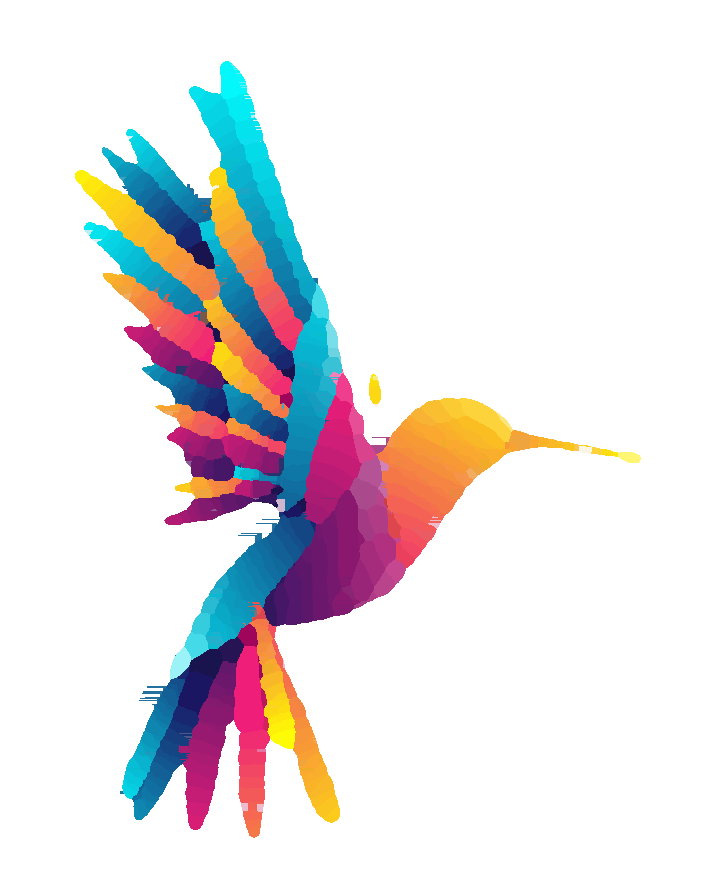The package provides other effects, such as `image_despeckle`, `image_reducenoise`, `image_motion_blur` and `image_emboss`.

## Drawing over images

It is possible to draw over the images making use of the `image_draw` function. You just need to set draw and then start adding base R elements, such as lines, text, markers, etc. Recall that you can get the width and height of the image with `image_info`, so you will know the axis limits.

``````# install.packages("magick")
library(magick)

# Get the size of the image before drawing
info <- image_info(r)
width <- info\$width
height <- info\$height

# Set an image_draw object
img <- image_draw(r)

abline(h = height / 2, col = 'blue', lwd = '10', lty = "dotted")
abline(v = width / 2, col = 'blue', lwd = '10', lty = "dotted")
rect(xleft = (width / 2) - 75, ybottom = (height / 2) - 75,
xright = (width / 2) + 75, ytop = (height / 2) + 75, border = "red", lwd = 5)
text(600, 600, "Text", cex = 5)

dev.off()``````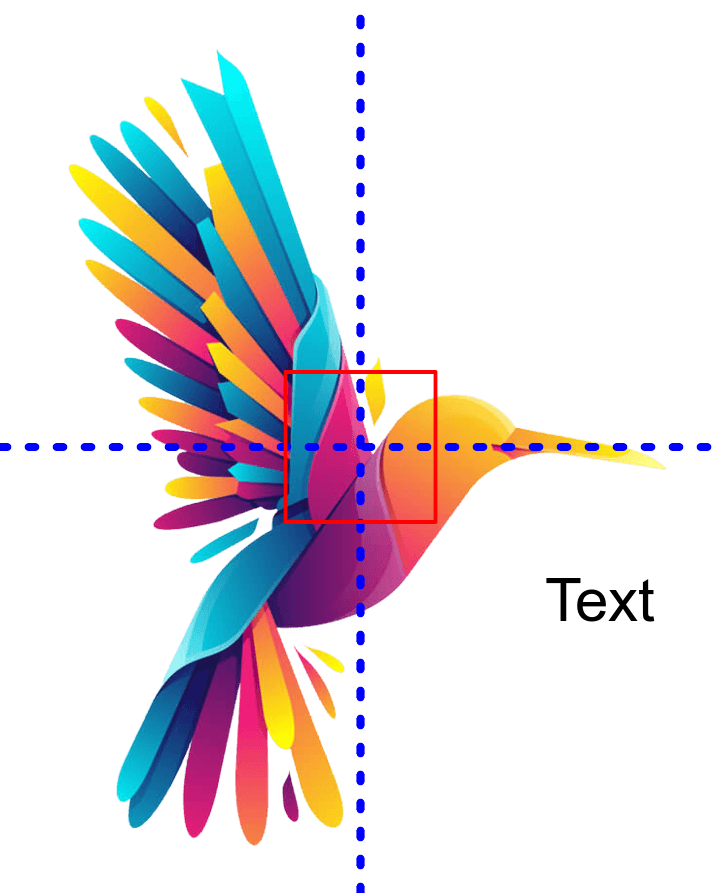## Composing images

Appending images side by side

It is possible to append several images with the `image_append` function. By default, the function will append the images side by side, but the images can also be stacked when `stack = TRUE`.

``````# install.packages("magick")
library(magick)

r2 <- image_read_svg('https://raw.githubusercontent.com/R-CoderDotCom/samples/main/tiger.svg', width = 500, height = 500)

# Join the images
r <- c(r1, r2)

# Append the images
image_append(r)``````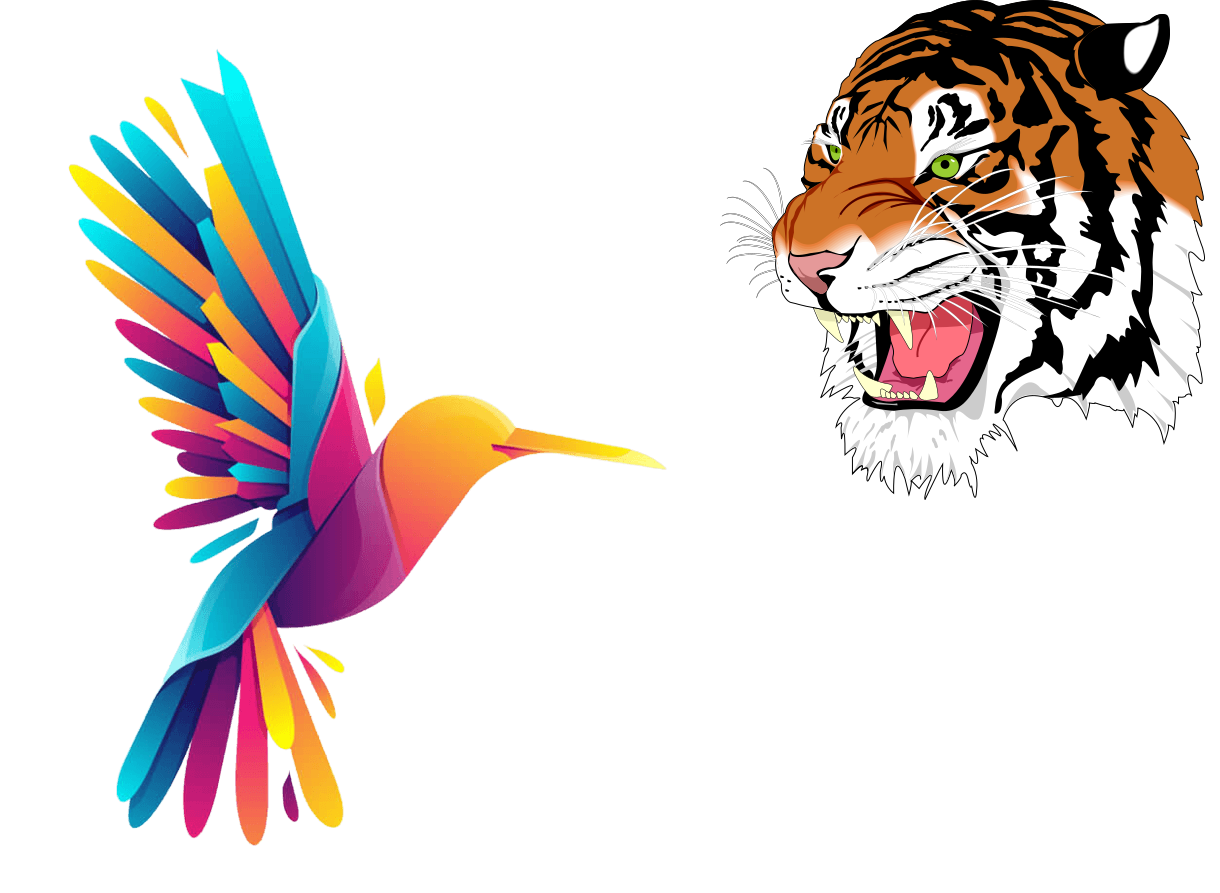Combining several images

You can combine several images with the `image_mosaic` function. An alternative is `image_flatten`, which will combine the images based on the size of the first image.

``````# install.packages("magick")
library(magick)

r2 <- image_read_svg('https://raw.githubusercontent.com/R-CoderDotCom/samples/main/tiger.svg', width = 500, height = 500)

# Join the images
r <- c(r1, r2)

# Combine the images
image_mosaic(r)``````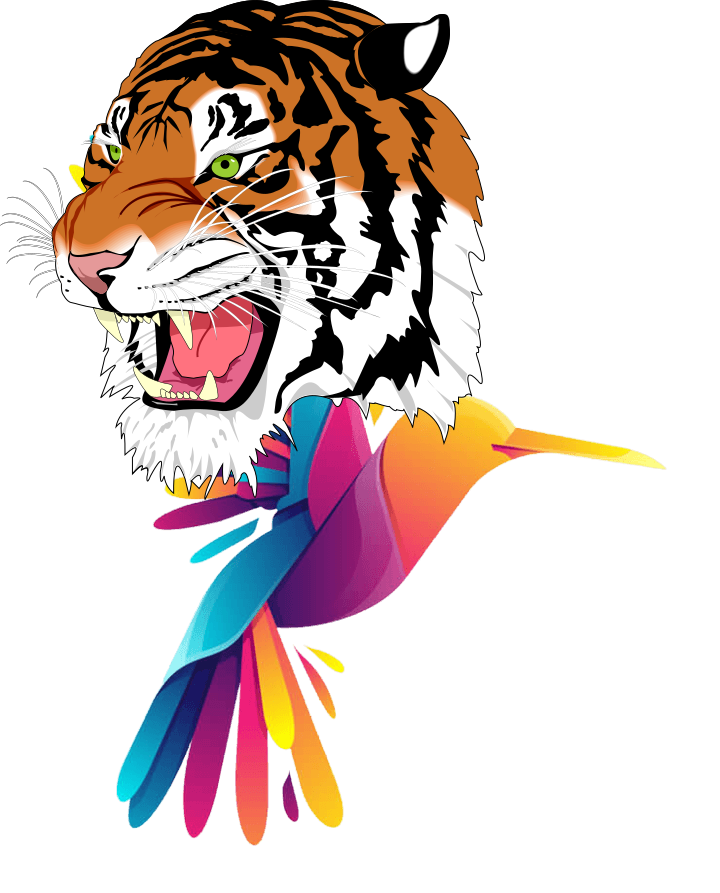Composing several images

The `image_composite` function composes an image over another using an operator. The default operator is `"atop"`, but you can write `compose_types()` in order to get the full list of available operators.

``````# install.packages("magick")
library(magick)

r2 <- image_read_svg('https://raw.githubusercontent.com/R-CoderDotCom/samples/main/tiger.svg', width = 500, height = 500)

# Compose the images
image_composite(image = r1, composite_image = r2)``````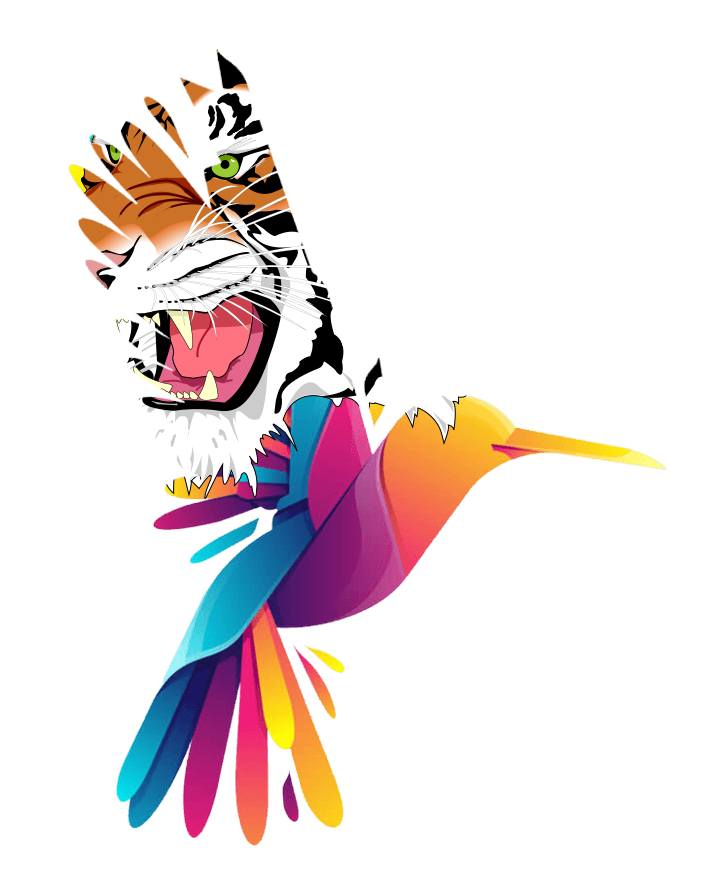Image over ggplot2 chart

A really interesting feature of the magick package is that allows adding the images over your charts. There are several alternatives to add them, but the best way is to use the `grid.raster` function from the `grid` package, as shown in the following example.

``````# install.packages("magick")
# install.packages("ggplot2")
# install.packages("grid")
library(magick)
library(ggplot2)
library(grid)

image_trim()

# ggplot2 plot
qplot(Sepal.Length, Sepal.Width, data = iris, geom = c("point", "smooth"))

# Image over plot at the center
grid.raster(r,
x = 0.5, y = 0.5,
width = unit(150, "points"))``````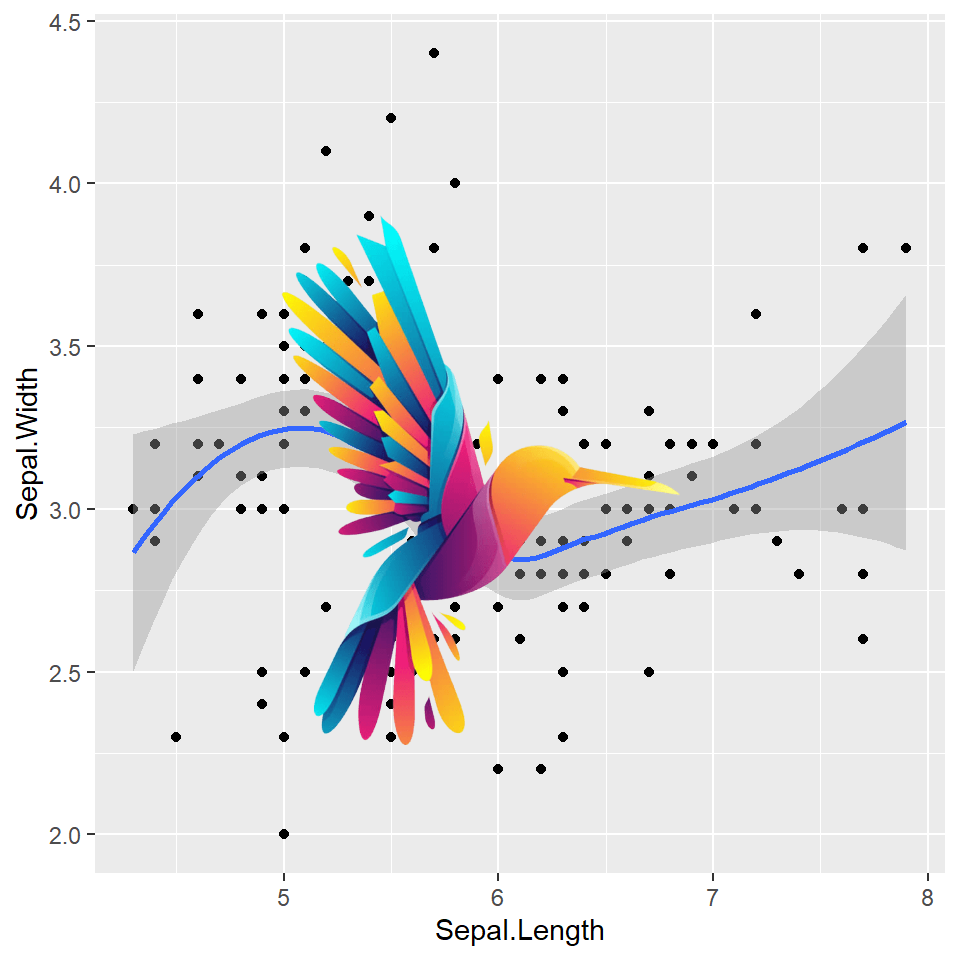Image over base R chart

Images can also be added to base R charts using the same function as the one used in the previous example.

``````# install.packages("magick")
# install.packages("grid")
library(magick)
library(grid)

image_trim()

# Plot
plot(1:10, pch = 16, cex = 5, col = rainbow(10))

# Image over plot at the center
grid.raster(r,
x = 0.5, y = 0.5,
width = unit(100, "points"))``````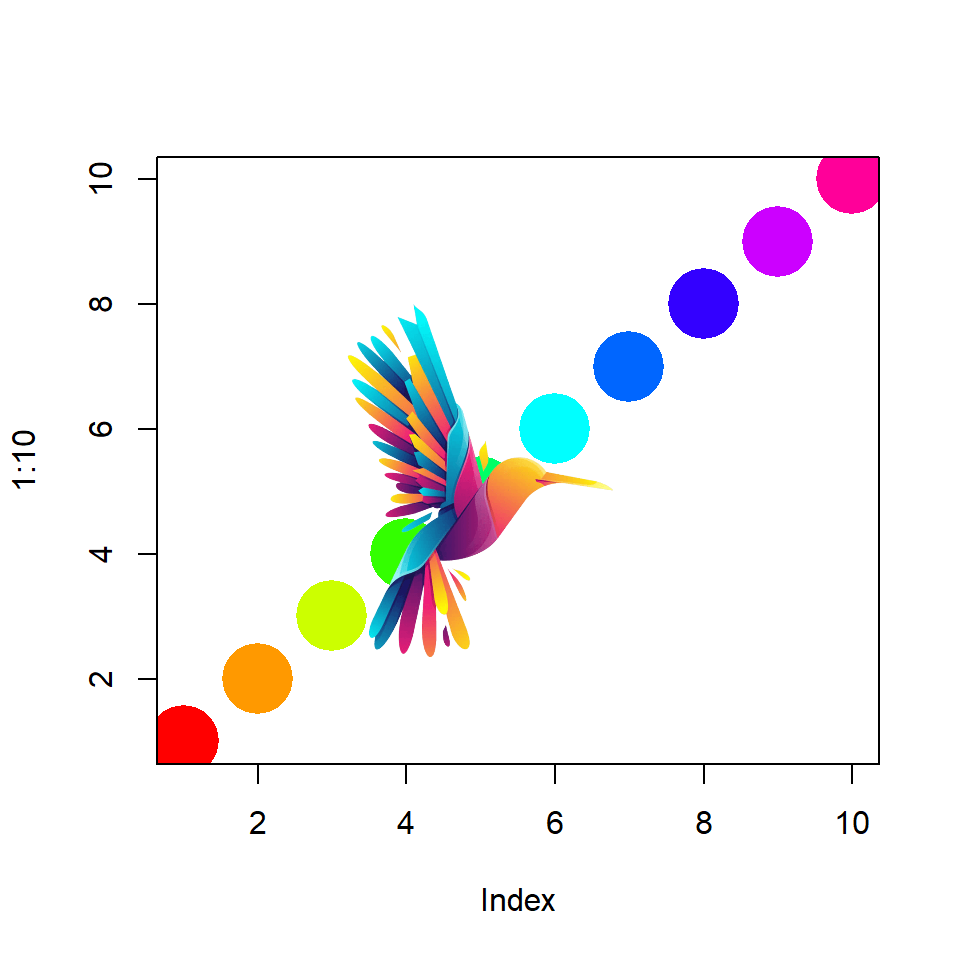## Animations

The `image_animate` function allows animating several images. By default the animation is at 10 frames per second but you can customize them with `fps`. You can also customize the disposal method with `dispose`, which possible values are `"background"`, `"previous"` and `"none"`.

``````# install.packages("magick")
library(magick)

r2 <- image_read_svg('https://raw.githubusercontent.com/R-CoderDotCom/samples/main/tiger.svg', width = 500, height = 500)

# Join the images
r <- c(r1, r2)

# Create a 1fps gif
image_animate(r, fps = 1, dispose = "previous")``````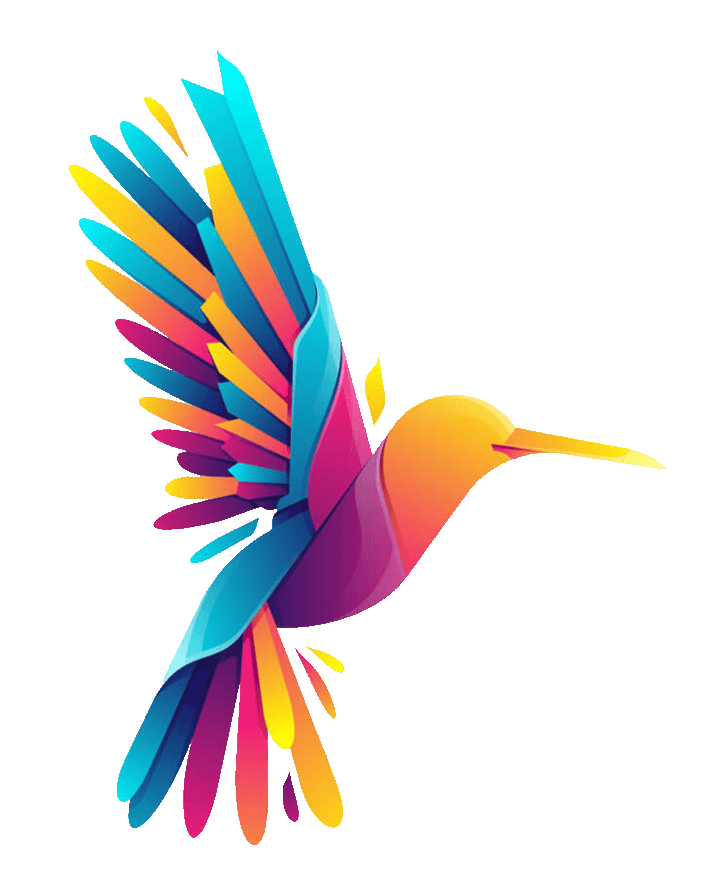With `image_morph` you can create a smooth transition between images. In the following example we are creating a transition between the normal image and the negative of the image.

``````# install.packages("magick")
library(magick)

image_negate()

# Join the images
r <- c(r1, r2)

# Animate with transition
r %>%
image_morph() %>%
image_animate(optimize = TRUE)``````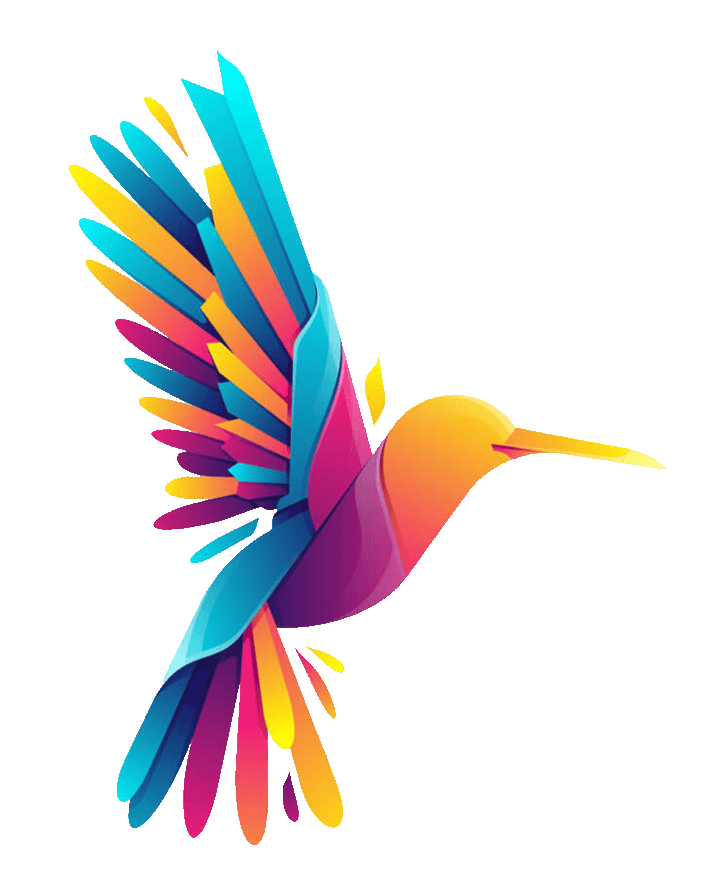You can also add gifs to your plots making use of several functions. You will need to use the `image_graph` function, create your plot, combine the gif and the plot with `image_composite` and then you can use the `image_animate` function to create the animation.

``````# install.packages("magick")
library(magick)
# install.packages("ggplot2")
library(ggplot2)

image_scale("250")

fig <- image_graph(width = 500, height = 500)

# Data
data <- data.frame(x = 1:12,
y = c(0, 100, 200, 457, 479, 550,
668, 800, 851, 880, 900, 1000))

ggplot(data, aes(x = x, y = y)) +
geom_text(aes(x = 4, y = 750, label = "magick package"), size = 10) +
geom_line(color = "blue", alpha = 0.9, linetype = 2)

dev.off()

# Composing the full image
out <- image_composite(fig, gif_image, offset = "+70+20", gravity = "southeast")

# Animation of the image
animation <- image_animate(out, fps = 10, optimize = TRUE)

# Show the image
print(animation, info = FALSE)``````